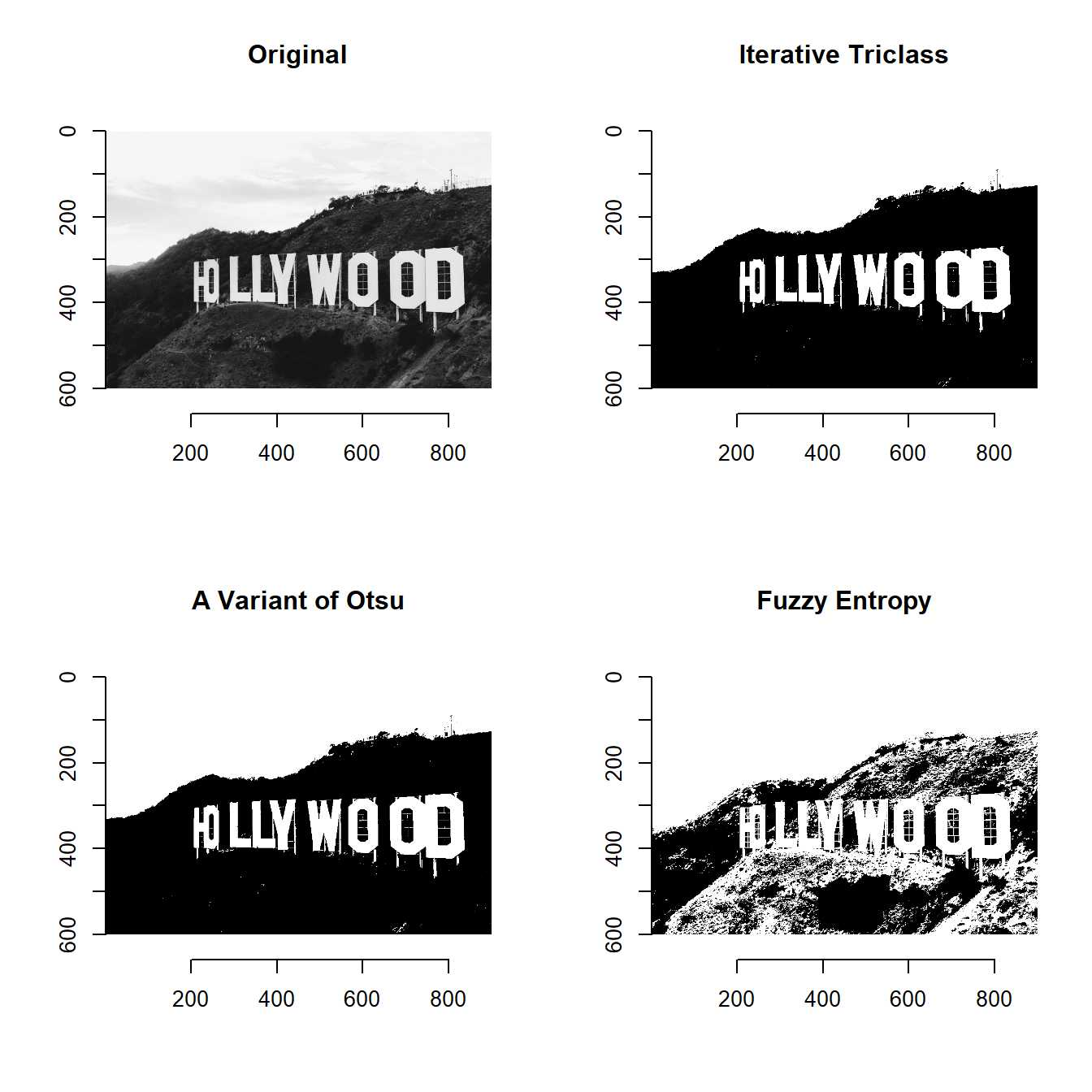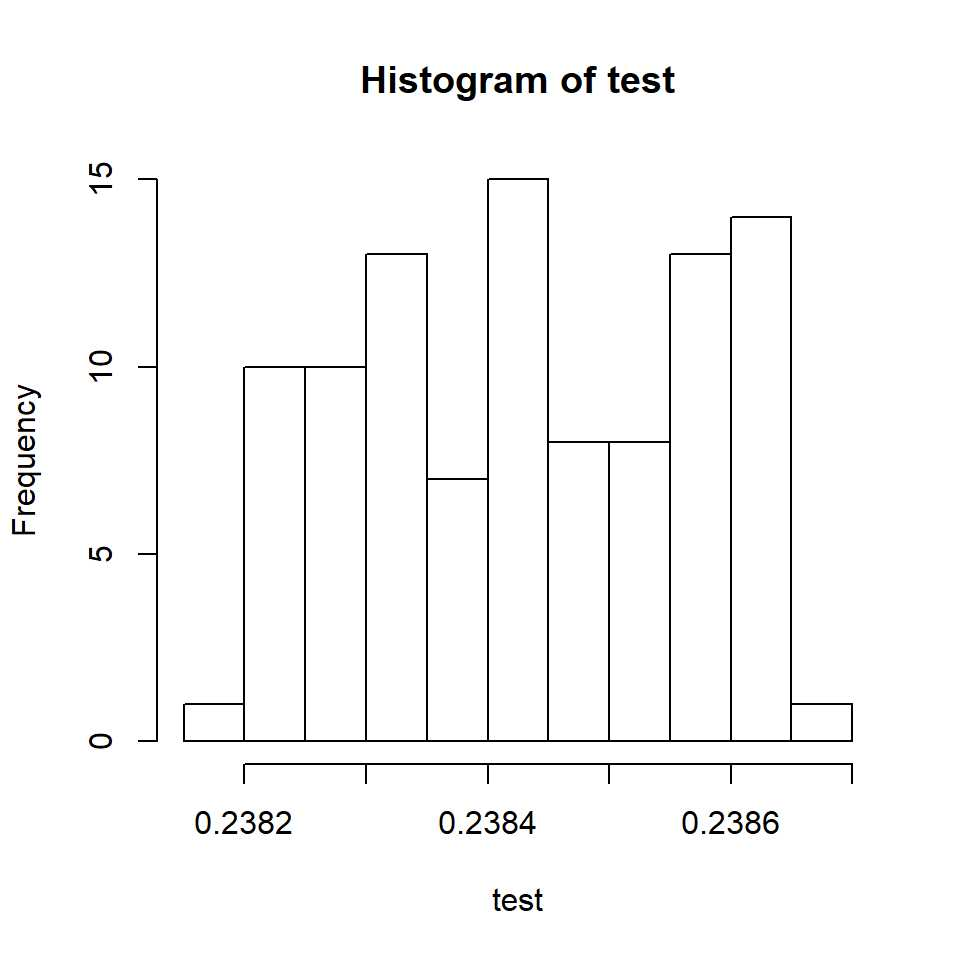Fuzzy Entropy Image Segmentation

Automatic thresholding is important for machine vision.

Otsu’s method is a novel algorithm and widely used for machine vision.

However, Otsu’s method is not suitable for some cases.

Fuzzy entropy image segmentation can be an alternative in such cases.

I implemented fuzzy entropy image segmentation (ThresholdFuzzy) by following .

You can confirm it by the code below.

library(devtools)
install_github("ShotaOchi/imagerExtra")
library(imagerExtra)
layout(matrix(1:4, 2, 2))
plot(a, main = "Original")
threshold(a) %>% plot(main = "A Variant of Otsu")
ThresholdTriclass(a) %>% plot(main = "Iterative Triclass")
ThresholdFuzzy(a) %>% plot(main = "Fuzzy Entropy")Note that ThresholdFuzzy uses a particle swarm optimization algorithm becase exaustive search is time consuming.

That’s why ThresholdFuzzy returns different value every time.

Let’s confirm it.

test <- sapply(1:100, function(x) ThresholdFuzzy(a, returnvalue = TRUE))
hist(test)You can reduce the variance by tuning the parameters of ThresholdFuzzy, although I believe the variance is sufficiently small.

 Linyi Li, Deren Li (2008). Fuzzy entropy image segmentation based on particle swarm optimization. Progress in Natural Science.